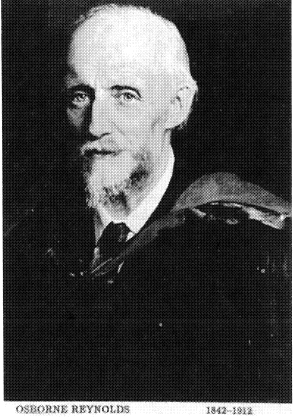## Pages

### Reynolds Analogy

During the later years of the 1800s several of the engineering challenges were related to the steam engines and their applications. One very important component of the steam engine is the Steam Boiler. The Steam boiler is the device in which heat transfer takes place. Several scientists studied and analyzed the heat transfer phenomenon that occurs between a solid and a liquid inter-phase. Up to this point it was known that the rate at which heat is transferred from a surface to a gas or vice versa is proportional to the difference in temperature between the two.Professor Osborne Reynolds however, questioned the assumption that amount of heat transferred from a solid to a fluid is independent of the fluid motion. Reynolds argued that the cooling effect of wind is very different from the cooling effect of still air and that this effect is so evident that any hypothesis for heat transfer which does not takes fluid motion into account will not be of any accuracy. Reynolds begins to discover that the heat transferred by a fluid from a surface at a different temperature from that of the fluid (Neglecting radiation) is proportional to the internal molecular diffusion of the fluid at and near the surface. Reynolds notes that the rate of internal diffusion is mainly dependent of two things: The natural internal diffusion of the fluid at rest (property of the fluid) and the rate of diffusion caused by fluid currents like eddies (due solely to fluid motion). Professor Osborne then developed a mathematical relationship for the heat transferred to a fluid based on the effect of these two (fluid at rest and fluid motion) Reynolds explains that when a fluid flows in a duct which surface temperature is higher than that of the fluid, there will be a velocity at which after this velocity, the temperature of the emerging gas is constant and independent of the fluid velocity. This velocity, as explained by Reynolds, is the same as that at which the frictional force on the duct due to the fluid motion is proportional to the velocity square. Reynolds then points out that the relationships then for heat transfer and momentum transfer (related to frictional forces) are therefore related and thus presents an analogy between momentum transfer and heat transfer. According to Professor Osborne, the velocity at which this happens is very small and therefore this analogy has a very practical use.

The Reynolds Analogy can be applied to both laminar and turbulent flows since this analogy takes into consideration any fluid movement. However, the analogy becomes even better for turbulent flows since the coefficient of turbulent exchange is larger in those cases making the kinematic viscosity and the thermometric conductivity negligible (This makes a direct proportionality between shearing stresses and heat transfer). For laminar flows, the Reynolds analogy holds better for gases since the kinematic viscosity and the thermal diffusivity are of about the same order. (Making a once again a direct proportionality between shearing stresses ad heat transfer)

The Reynolds Analogy holds true for flows in which the temperature profile and the velocity profile are “similar” (including boundary conditions) and thus the heat and momentum are transported at the same rate.  These conditions are met if: 1 the pressure gradient in the direction of the flow is zero, 2 the coefficient of turbulent exchange thermal is equal to the coefficient of turbulent exchange for the momentum (turbulent Prandtl number approximately equal to 1) or 3 if the kinematic viscosity is approximately equal to the thermal diffusivity (Prandtl number is approximately equal to 1). Therefore, for external flow (Laminar or turbulent), the Reynolds analogy holds true since the pressure gradient in the direction of the flow is usually zero. For internal flows, this is not the case, (dp/dx does not equals zero). However, for internal fully developed turbulent flows the pressure and temperature gradient along the flow direction is approximately constant. Therefore for internal, fully developed, turbulent flow, the momentum and heat transfer equation are similar if the conditions 2 and 3 discussed above are met.

1.2.